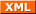# Christoph's last Weblog entries

### Entries tagged "ictf".

22nd March 2018

This is FAUST playing CTF again, this time iCTF. We somehow managed to score an amazing 5th place.

Team: FAUST
Crew: izibi, siccegge
Files: spiderman

spider is a patched python interpreter. man is a pyc but with different magic values (explaining the patched python interpreter for now). Plain decompiling failes due to some (dead) cruft code at the beginning of all methods. can be patched away or you do more manual disassembling.

Observations:

• does something with RSA
• public exponent is slightly uncommon ($$2^{16}+3$$ instead of $$2^{16}+1$$) but that should be fine.
• uses openssl prime -generate to generate the RSA key. Doesn't use -safe but should also be fine for RSA purposes
• You need to do a textbook RSA signature on a challenge to get the flag

Fine so far nothing obvious to break. When interacting with the service, you will likely notice the Almost Equal function in the Fun menu. According to the bytecode, it takes two integers $$a$$ and $$b$$ and outputs if $$a = b \pm 1$$, but looking at the gameserver traffic, these two numbers are also considered to be almost equal:

$$a = 33086666666199589932529891 \\ b = 35657862677651939357901381$$

So something's strange here. Starting the spider binary gives a python shell where you can play around with these numbers and you will find that a == b - 1 will actually result in True. So there is something wrong with the == operator in the shipped python interpreter, however it doesn't seem to be any sort of overflow. Bit representation also doesn't give anything obvious. Luky guess: why the strange public exponent? let's try the usual here. and indeed $$a = b - 1 \pmod{2^{16}+1}$$. Given this is also used to compare the signature on the challenge this becomes easily bruteforceable.

#!/usr/bin/env python3

import nclib, sys
from random import getrandbits

e = 2**16+3 # exponent
w = 2**16+1 # wtf

nc = nclib.Netcat((sys.argv, 20005), udp=False, verbose=True)
nc.recv_until(b'4) Exit\n')

nc.recv_until(b'What do you want to read?\n')
nc.send(sys.argv.encode() + b'\n')

nc.recv_until(b'solve this:\n')
modulus, challenge = map(int, nc.recv_until(b'\n').decode().split()[:2])
challenge %= w

# Starting at 0 would also work, but using large random numbers makes
# it less obvious that we only bruteforce a small set of numbers
while (pow(answer, e, modulus)) % w != challenge: